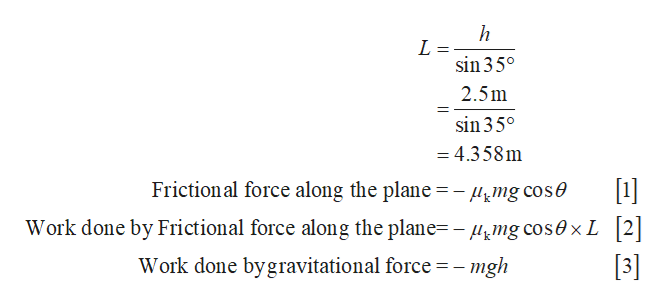Question
13 views

A 60.0 kg skier with an initial speed of 11.0 m/s coasts up a 2.50 m high rise as shown in Figure 6.23. Find his final speed at the top, given that the coefficient of friction between her skis and the snow is 0.0800. (Hint: Find the distance traveled up the incline assuming a straight-line path as shown in the figure.)
m/s

Figure 6.23
check_circle

Step 1

The below figure represents the distance travelled up the plane by a 60kg skier with an initai speed of 11.0m/s which is 2.50m high rise .

Step 2

Write the expression for distance travelle...help_outlineImage Transcriptioncloseh L = sin 35° 2.5m sin 35° = 4.358m Frictional force along the plane =- mg cose  Work done by Frictional force along the plane= -mg COS0XL 2 Work done bygravitational force =-mgh  fullscreen

### Want to see the full answer?

See Solution

#### Want to see this answer and more?

Solutions are written by subject experts who are available 24/7. Questions are typically answered within 1 hour.*

See Solution
*Response times may vary by subject and question.
Tagged in
SciencePhysics

### Kinematics